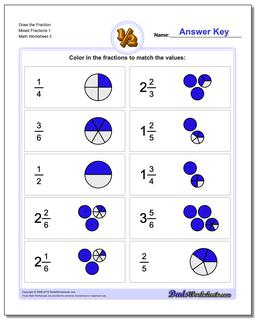PLEASE GO BACK AND USE THE BIG BLUE 'PRINT' BUTTON ON THE PAGE TO PRINT THE WORKSHEET CORRECTLY!Sorry for the trouble! The browser won't print the embedded worksheet PDF directly using the normal 'Print' command in the file menu, so you need to click the big 'Print' button to send just the worksheet and not the surrounding page to the printer.

# Math Worksheets: Graphic Fractions: Graphic Fractions: Draw the Fraction Mixed Fractions 1 (Third Worksheet)## Draw the Fraction Mixed Fractions 1 (Third Worksheet)

Property Value
DescriptionDraw the Fraction Mixed Fractions 1: For these problems, kids are given a simple fraction and asked to draw a pie chart representation of the value completely independently; unlike the worksheets from the prior section, these worksheets do not include the pre-divided fraction shapes as hints. Initial worksheets start out with basic fractions, and later sheets introduce mixed fractions. (Third Worksheet)
Resource TypeWorksheet